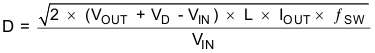SLVSCN9B December   2014  – June 2020

PRODUCTION DATA.

1. Features
2. Applications
3. Description
1.     Device Images
4. Revision History
5. Pin Configuration and Functions
6. Specifications
7. Detailed Description
1. 7.1 Overview
2. 7.2 Functional Block Diagram
3. 7.3 Feature Description
4. 7.4 Device Functional Modes
8. Application and Implementation
1. 8.1 Application Information
2. 8.2 Typical Application
9. Power Supply Recommendations
10. 10Layout
11. 11Device and Documentation Support
12. 12Mechanical, Packaging, and Orderable Information

• PWP|14
• PWP|14

#### 8.2.2.1 Determining the Duty Cycle

The TPS61175-Q1 has a maximum worst case duty cycle of 89% and a minimum on time of 60 ns. These two constraints place limitations on the operating frequency that can be used for a given input to output conversion ratio. The duty cycle at which the converter operates is dependent on the mode in which the converter is running. If the converter is running in discontinuous conduction mode (DCM), where the inductor current ramps to zero at the end of each cycle, the duty cycle varies with changes to the load much more than it does when running in continuous conduction mode (CCM). In continuous conduction mode, where the inductor maintains a dc current, the duty cycle is related primarily to the input and output voltages as computed below:

Equation 3.In discontinuous mode the duty cycle is a function of the load, input and output voltages, inductance and switching frequency as computed below:

Equation 4.All converters using a diode as the freewheeling or catch component have a load current level at which they transition from discontinuous conduction to continuous conduction. This is the point where the inductor current just falls to zero. At higher load currents, the inductor current does not fall to zero but remains flowing in a positive direction and assumes a trapezoidal wave shape as opposed to a triangular wave shape. This load boundary between discontinuous conduction and continuous conduction can be found for a set of converter parameters as follows.

Equation 5.For loads higher than the result of the equation above, the duty cycle is given by Equation 3 and for loads less than the results of Equation 4, the duty cycle is given in Equation 5. For Equation 3 through Equation 5, the variable definitions are as follows.

• VOUT is the output voltage of the converter in V
• VD is the forward conduction voltage drop across the rectifier or catch diode in V
• VIN is the input voltage to the converter in V
• IOUT is the output current of the converter in A
• L is the inductor value in H
• fSW is the switching frequency in Hz

Unless otherwise stated, the design equations that follow assume that the converter is running in continuous mode.